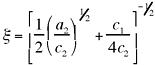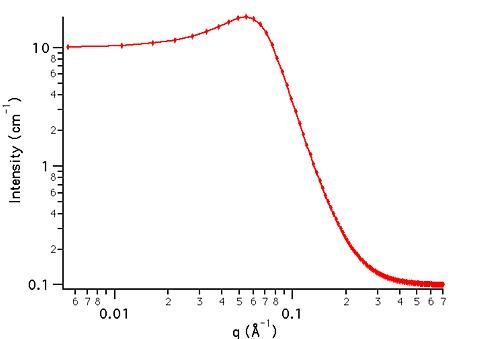MODEL

TeubnerStrey

AUTHOR/MODIFICATION

Steve Kline 06 NOV 1998

Alan Munter 08 July 1999, converted to Java

APPROVED FOR DISTRIBUTION

DESCRIPTION

This function calculates the scattered intensity of a two-component system using the Teubner-Strey model.

VARIABLES

Input Variables (default values):

Parameter Variable Value
0Scale (a2)0.1
1c1-30.0
2c25000.0
3Incoherent Background (cm-1)0.0

USAGE NOTES

The returned value is on an arbitrary scale.

the function calculated is:where the correlation length (the dispersion of d) is:and the quasi-periodic repeat distance is:Parameter (a2) acts as a scale factor, taking note that it is in the denominator and will affect the magnitude of c1 and c2.

REFERENCE

Teubner, M; Strey, R. J. Chem. Phys., 1987, 87, 3195.

Schubert, K-V.; Strey, R.; Kline, S. R.; and E. W. Kaler J. Chem. Phys., 1994, 101, 5343.

TEST DATASET

This example dataset is produced by calculating the TeubnerStrey model using data points, qmin = 0.001 Å-1, qmax = 0.7 (Å-1) and the above default parameter values.